## Example Questions

### Example Question #1 : How To Subtract Fractions

Which fraction has a value greater than that of X?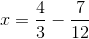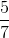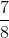Explanation:

First, start by putting changing the fractions in the equation so that they have the same denominator.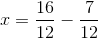Then, you can easily subtract the numerators. The denominator stays the same at this point: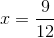At this point, you can reduce the fraction by dividing the numerator and the denominator by: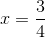Then, to easily compare the fraction to the answer choices, you can change the fraction to a decimal: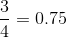By changing the answer choices to decimals as well, you can easily figure out which value is greater than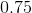: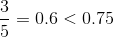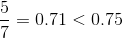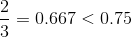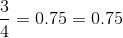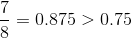, sois the correct answer.